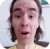FreeKB - if elsif else statements in Perl
if elsif else statements in PerlAn if elsif statement in Perl has the following structure.

``````if (comparison) {
--do something--
}
elsif (comparison) {
--do something--
}
else {
--do something
}``````

For example.

``````if (\$foo == 10) {
print "foo equals 10.";
}
elsif (\$foo == 20) {
print "foo equals 20.";
}
else {
print "foo does not equal 10 or 20.";
}``````

Following are common if statements.

 Equals if (\$foo == \$bar) # integer if (\$foo eq \$bar) # string Does not equal if (\$foo != \$bar) # integer if (\$foo ne \$bar) # string Greater than if (\$foo > \$bar) # integer if (\$foo gt \$bar) # string Less than if (\$foo < \$bar) # integer if (\$foo lt \$bar) # string Greater than or equal to if (\$foo >= \$bar) # integer if (\$foo ge \$bar) # string Less than or equal to if (\$foo <= \$bar) # integer if (\$foo le \$bar) # string If file or directory exists if ( -e "/path/to/file.txt") if ( -e "/path/to/directory") If file or directory does not exist if ( not -e "/path/to/file.txt") if ( not -e "/path/to/directory") If variable is defined if (defined \$foo) If variable is not defined if (not defined \$foo) If variable is empty (null) if (\$foo) If variable is not empty (not null) if (not \$foo) If array is empty (null) if (@foo == 0) If array is not empty (not null) if (@foo != 0) If variable contains if (\$foo =~ /bar/) If variable does not contain if (\$foo !~ /bar/) If array contains if (grep(/bar/, @foo )) If array does not contain if (not grep(/bar/, @foo )) If array contains exact match if (grep(/^bar\$/, @foo )) If array does not contain exact match if (not grep(/^bar\$/, @foo )) If hash contains if (grep(/bar/, %hash )) If hash does not contain if (not grep(/bar/, %hash )) If file contains / does not contain refer to this article If string begins with if (\$foo =~ /^bar/) If string does not begin with if (\$foo !~ /^bar/) If string ends with if (\$foo =~ /bar\$/) If string does not end with if (\$foo !~ /bar\$/)

If directory is empty (care of this Stack Overflow post).

``````sub directory_is_empty {
my \$dirname = shift;
opendir(my \$dh, \$dirname);
return scalar(grep { \$_ ne "." && \$_ ne ".." } readdir(\$dh)) == 0;
}``````

Use the subroutine.

``````if (directory_is_empty("/path/to/directory")) {
....
}``````

Ignore case

The i option can be used to ignore case.

``if (\$foo =! /bar/i)``

Multiple conditions

Multiple conditions can be evaluated by separating each condition with and or or.

``if (--condition-- and --condition-- or --condition--)``

More precise conditions can be strung together by evaluating certain conditions together. The possible arrangement of evaluations is literally limitless.

``if( (--condition and --condition) or --condition)``

Regular Expressions

Following are commonly used regular expressions.

 Single integer (0 through 9) [0-9] Single integer followed by anything [0-9]* Anything followed by a single integer *[0-9] Single lower case alpha character [a-z] Single lower case alpha character followed by anything [a-z]* Anything followed by single lower case alpha character *[a-z] Single upper case character [A-Z] Single upper case alpha character followed by anything [A-Z]* Anything followed by single upper case alpha character *[A-Z] Single alpha character [a-zA-Z] Single alpha character followed by anything [a-zA-Z]* Anything followed by alpha character *[a-zA-Z] Any alpha-numeric character [0-9a-zA-Z] Any alpha-numeric character followed by anything [0-9a-zA-Z]* Anything followed by any alpha-numeric character *[0-9a-zA-Z] Any single character except new line . Any single character except new line followed by anything .* Space, tab, or newline \s Not a space, tab, or newline \S

We will never share your name or email with anyone. Enter your email if you would like to be notified when we respond to your comment.

Please enter 22544 in the box below so that we can be sure you are a human.